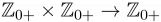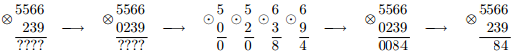## Problem B. Binary Operation

Consider a binary operation ⊙ defined on digits 0 to 9, ⊙ : {0, 1, ..., 9} × {0, 1, ..., 9} → {0, 1, ..., 9}, such that 0 ⊙ 0 = 0.

A binary operator ⊗ is a generalization of ⊙ to the set of non-negative integers, ⊗ :$\displaystyle \mathbb{Z}_{0+} \times \mathbb{Z}_{0+} \rightarrow \mathbb{Z}_{0+}$. The result of ab is defined in the following way: if one of the numbers a and b has fewer digits than the other in decimal notation, then append leading zeroes to it, so that the numbers are of the same length; then apply the operation ⊙ digit-wise to the corresponding digits of a and b.Example. If ab = ab mod 10, then 5566 ⊗ 239 = 84.

Let us define ⊗ to be left-associative, that is, abc is to be interpreted as (ab) ⊗ c.

Given a binary operation ⊙ and two non-negative integers a and b, calculate the value of a ⊗ (a + 1) ⊗ (a + 2) ⊗ ... ⊗ (b - 1) ⊗ b.

### Input

The first ten lines of the input contain the description of the binary operation ⊙. The i-th line of the input contains a space-separated list of ten digits - the j-th digit in this list is equal to (i - 1)⊙(j - 1).
The first digit in the first line is always 0.
The eleventh line of the input contains two non-negative integers a and b (0 ≤ a ≤ b ≤ 1018)

### Output

Output a single number - the value of a ⊗ (a + 1) ⊗ (a + 2) ⊗ ... ⊗ (b - 1) ⊗ b without extra leading zeroes.

### Sample Input

0 1 2 3 4 5 6 7 8 9
1 2 3 4 5 6 7 8 9 0
2 3 4 5 6 7 8 9 0 1
3 4 5 6 7 8 9 0 1 2
4 5 6 7 8 9 0 1 2 3
5 6 7 8 9 0 1 2 3 4
6 7 8 9 0 1 2 3 4 5
7 8 9 0 1 2 3 4 5 6
8 9 0 1 2 3 4 5 6 7
9 0 1 2 3 4 5 6 7 8
0 10


### Sample Output

15


Point Value: 20 (partial)
Time Limit: 2.00s
Memory Limit: 64M